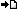RegisterMember LoginForgot Password ?? PHP , ASP , ASP.NET, VB.NET, C#, Java , jQuery , Android , iOS , Windows Phone

## Registered : 109,007

 HOME > PHP Manual > Operators - Operators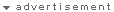## ลองใช้ค้นหาข้อมูล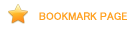# Operators

An operator is something that you feed with one or more values (or expressions, in programming jargon) which yields another value (so that the construction itself becomes an expression). So you can think of functions or constructions that return a value (like print) as operators and those that return nothing (like echo) as any other thing.

There are three types of operators. Firstly there is the unary operator which operates on only one value, for example ! (the negation operator) or ++ (the increment operator). The second group are termed binary operators; this group contains most of the operators that PHP supports, and a list follows below in the section Operator Precedence.

The third group is the ternary operator: ?:. It should be used to select between two expressions depending on a third one, rather than to select two sentences or paths of execution. Surrounding ternary expressions with parentheses is a very good idea.

## Operator Precedence

The precedence of an operator specifies how "tightly" it binds two expressions together. For example, in the expression 1 + 5 * 3, the answer is 16 and not 18 because the multiplication ("*") operator has a higher precedence than the addition ("+") operator. Parentheses may be used to force precedence, if necessary. For instance: (1 + 5) * 3 evaluates to 18. If operator precedence is equal, left to right associativity is used.

The following table lists the precedence of operators with the highest-precedence operators listed at the top of the table. Operators on the same line have equal precedence, in which case their associativity decides which order to evaluate them in.
Operator Precedence
non-associative clone new clone and new
left [ array()
non-associative ++ -- increment/decrement
non-associative ~ - (int) (float) (string) (array) (object) (bool) @ types
non-associative instanceof types
right ! logical
left * / % arithmetic
left + - . arithmetic and string
left << >> bitwise
non-associative < <= > >= <> comparison
non-associative == != === !== comparison
left & bitwise and references
left ^ bitwise
left | bitwise
left && logical
left || logical
left ? : ternary
right = += -= *= /= .= %= &= |= ^= <<= >>= assignment
left and logical
left xor logical
left or logical
left , many uses

Left associativity means that the expression is evaluated from left to right, right associativity means the opposite.

Example #1 Associativity

``` <?php\$a = 3 * 3 % 5; // (3 * 3) % 5 = 4\$a = true ? 0 : true ? 1 : 2; // (true ? 0 : true) ? 1 : 2 = 2\$a = 1;\$b = 2;\$a = \$b += 3; // \$a = (\$b += 3) -> \$a = 5, \$b = 5?> ```
Use parentheses to increase readability of the code.

Note: Although = has a lower precedence than most other operators, PHP will still allow expressions similar to the following: if (!\$a = foo()), in which case the return value of foo() is put into \$a.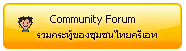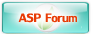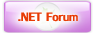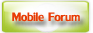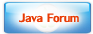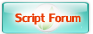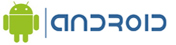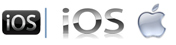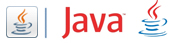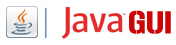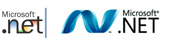Free Tutorial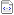สอน Google Maps Apiสอน Windows Serviceสอน Entity Frameworkสอน Androidสอน Java เขียน JavaJava GUI Swingสอน JSP (Web App)iOS (iPhone,iPad)Windows PhoneWindows AzureWindows StoreLaravel FrameworkYii PHP Frameworkสอน jQueryสอน jQuery กับ Ajaxสอน PHP OOP (Vdo)Ajax Tutorials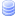SQL Tutorialsสอน SQL (Part 2)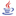JavaScript TutorialJavascript TipsVBScript TutorialVBScript ValidationMicrosoft Access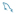MySQL Tutorials-- Stored ProcedureMariaDB Database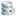SQL Server TutorialSQL Server 2005SQL Server 2008SQL Server 2012-- Stored ProcedureOracle Database-- Stored ProcedureSVN (Subversion)แนวทางการทำ SEOปรับแต่งเว็บให้โหลดเร็วสุดยอด Source Code V2.0แจ้งชำระเงิน/โอนเงินลองค้นหาสิ่งที่ต้องการLoad balance : Server 05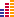© www.ThaiCreate.Com. 2003-2023 All Rights Reserved. ไทยครีเอทบริการ จัดทำดูแลแก้ไข Web Application ทุกรูปแบบ (PHP, .Net Application, VB.Net, C#) [Conditions Privacy Statement] ติดต่อโฆษณา 081-987-6107 อัตราราคา คลิกที่นี่